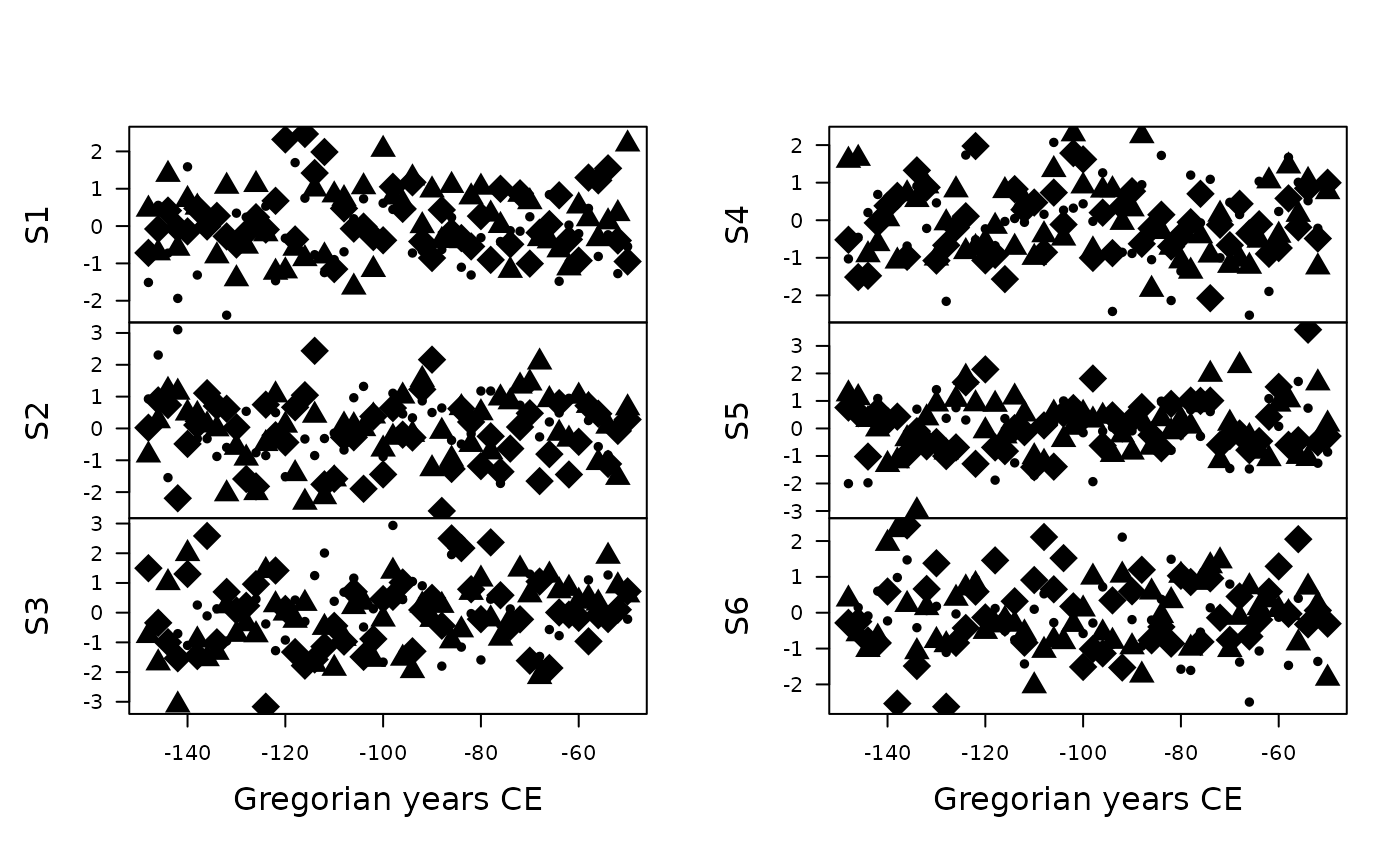Plot Time Series

## Usage

# S4 method for TimeSeries,missing
plot(
x,
facet = c("multiple", "single"),
calendar = getOption("aion.calendar"),
panel = graphics::lines,
flip = FALSE,
ncol = NULL,
xlab = NULL,
ylab = NULL,
main = NULL,
sub = NULL,
ann = graphics::par("ann"),
axes = TRUE,
frame.plot = axes,
panel.first = NULL,
panel.last = NULL,
...
)

## Arguments

x

A TimeSeries object.

facet

A character string specifying whether the series should be plotted separately (with a common time axis) or on a single plot? It must be one of "multiple" or "single". Any unambiguous substring can be given.

calendar

A TimeScale object specifying the target calendar (see calendar()).

panel

A function in the form function(x, y, ...) which gives the action to be carried out in each panel of the display. The default is graphics::lines().

flip

A logical scalar: should the y-axis (ticks and numbering) be flipped from side 2 (left) to 4 (right) from series to series when facet is "multiple"?

ncol

An integer specifying the number of columns to use when facet is "multiple". Defaults to 1 for up to 4 series, otherwise to 2.

xlab, ylab

A character vector giving the x and y axis labels.

main

A character string giving a main title for the plot.

sub

A character string giving a subtitle for the plot.

ann

A logical scalar: should the default annotation (title and x and y axis labels) appear on the plot?

axes

A logical scalar: should axes be drawn on the plot?

frame.plot

A logical scalar: should a box be drawn around the plot?

panel.first

An an expression to be evaluated after the plot axes are set up but before any plotting takes place. This can be useful for drawing background grids.

panel.last

An expression to be evaluated after plotting has taken place but before the axes, title and box are added.

...

Further parameters to be passed to panel (e.g. graphical parameters).

## Value

plot() is called for its side-effects: it results in a graphic being displayed. Invisibly returns x.

graphics::plot()

Other plotting tools: image(), year_axis()

N. Frerebeau

## Examples

## Create 6 time-series of 50 observations
## Sampled every two years starting from 2000 BP
X <- series(
object = matrix(rnorm(300), nrow = 50, ncol = 6),
time = seq(2000, by = -2, length.out = 50),
calendar = BP()
)

## Multiple
plot(X) # Default calendar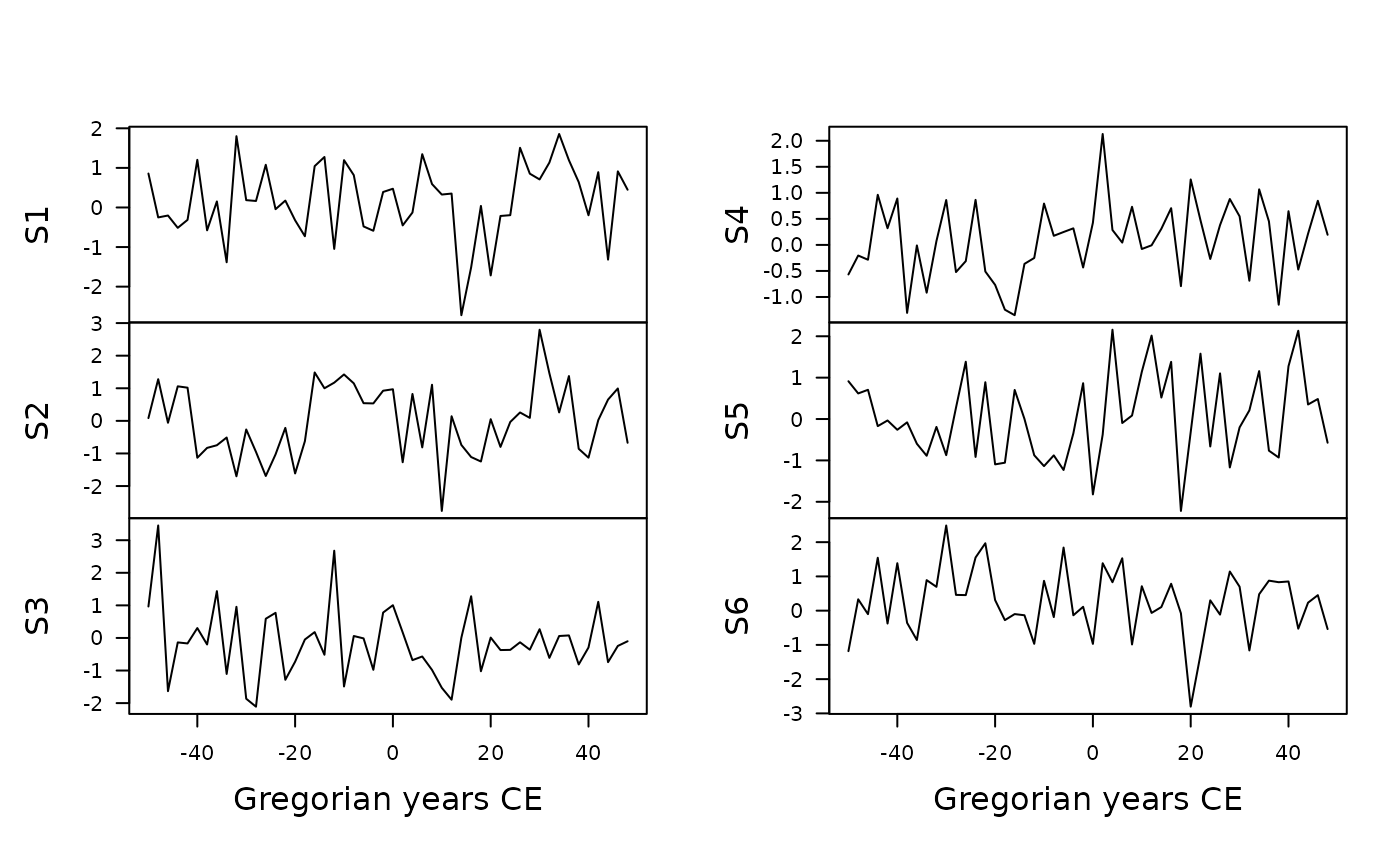plot(X, calendar = BP(), flip = TRUE) # BP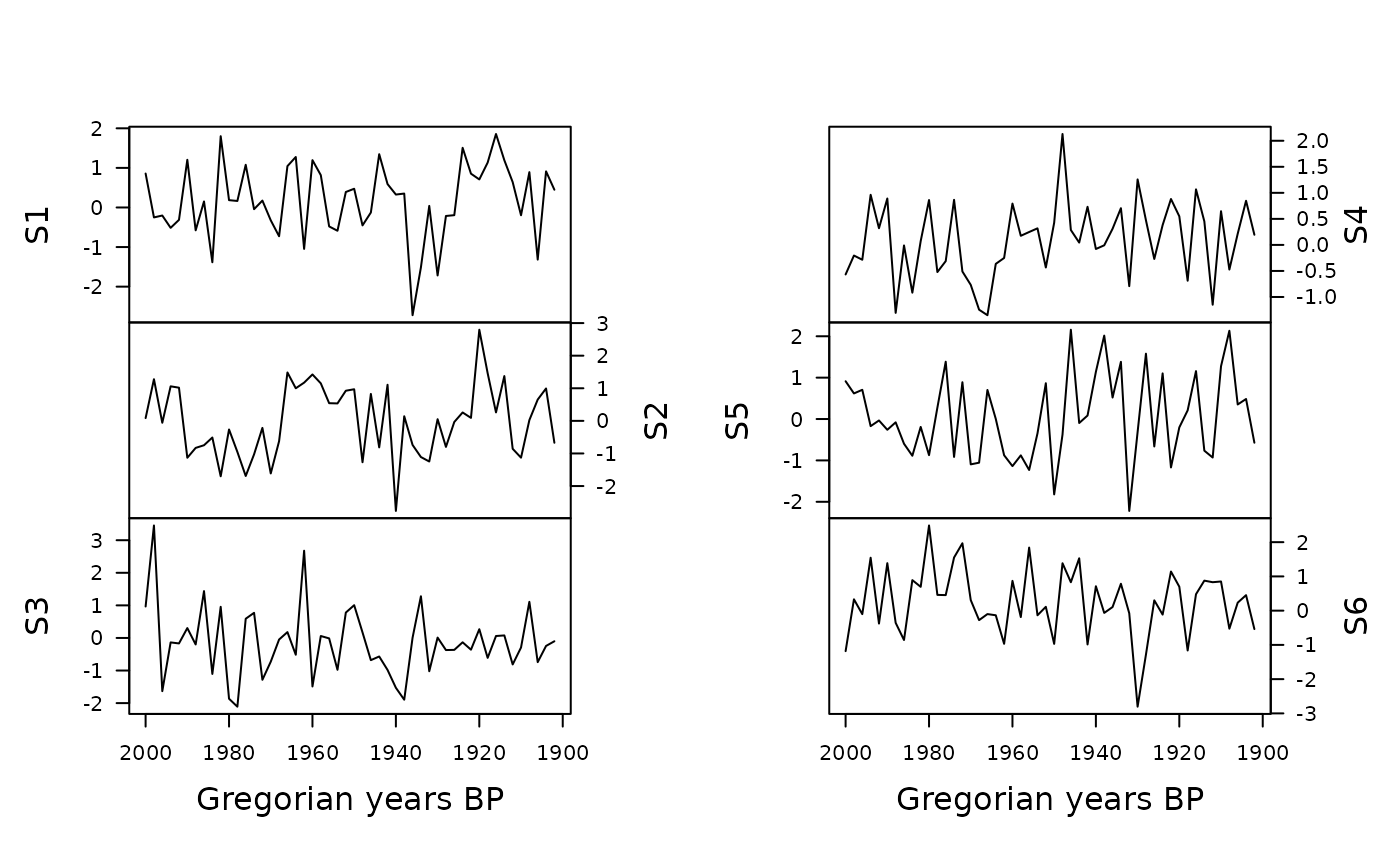plot(X, calendar = b2k(), ncol = 1) # b2k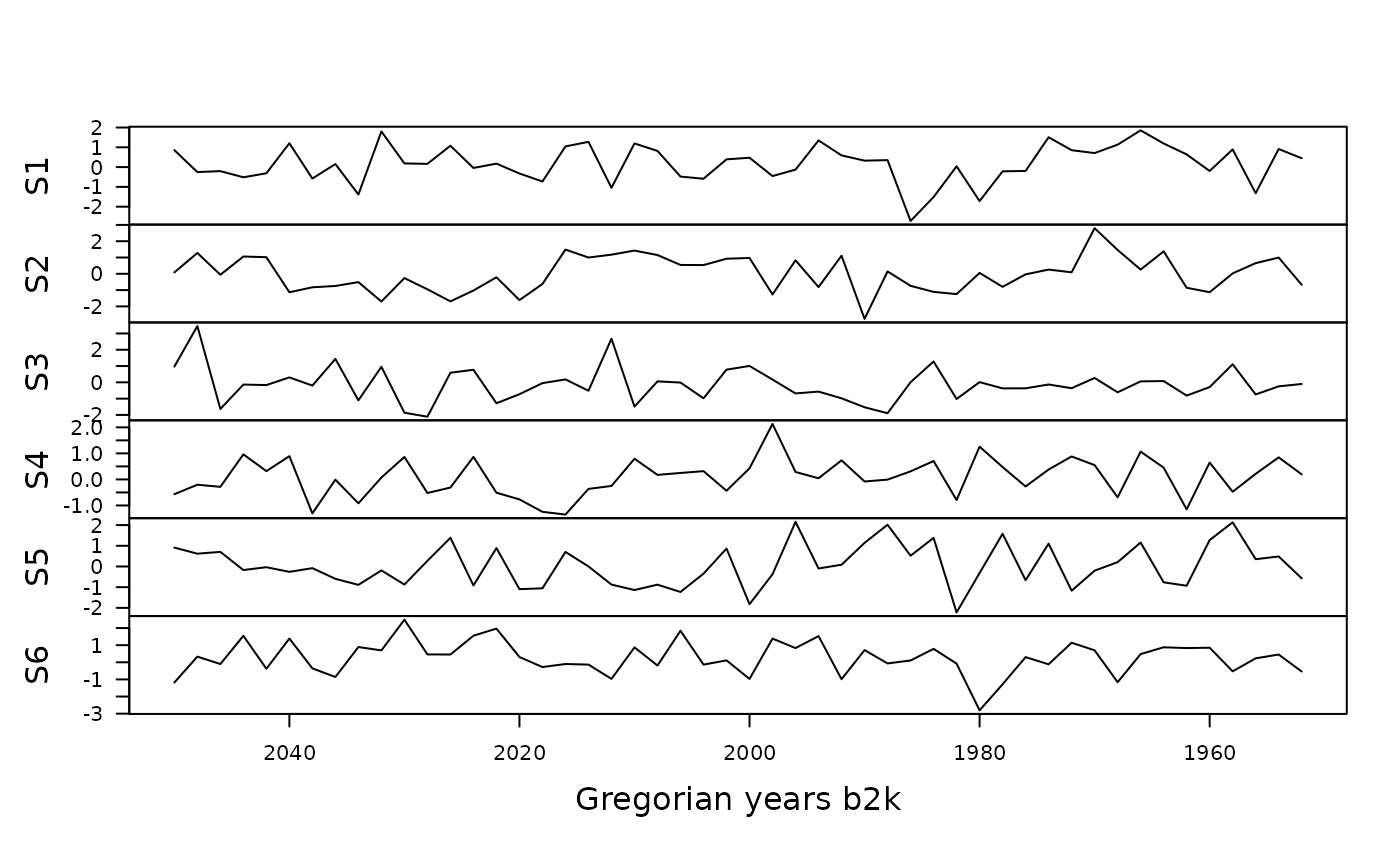## Single
plot(X, facet = "single") # CE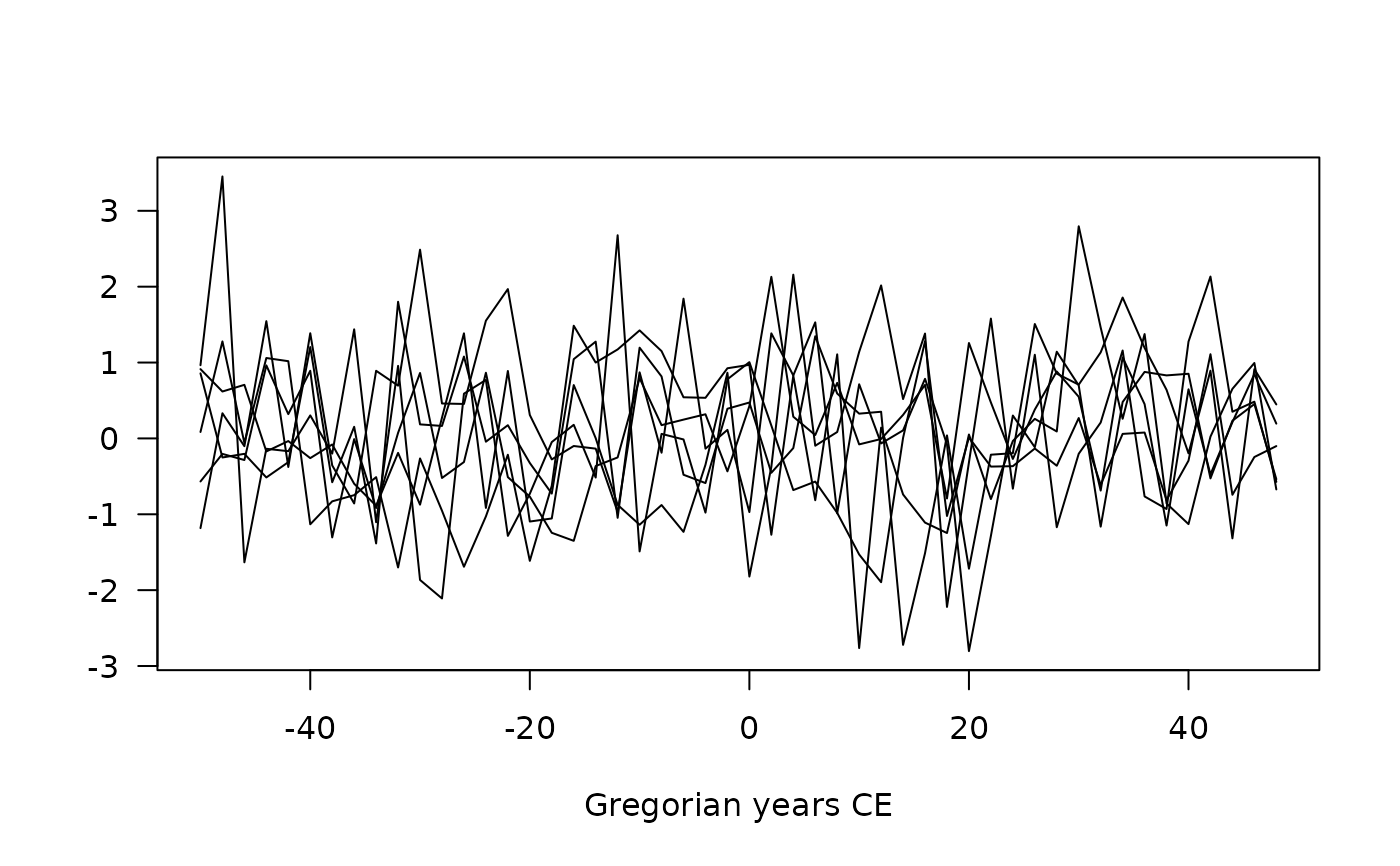plot(X, facet = "single", calendar = BP()) # BP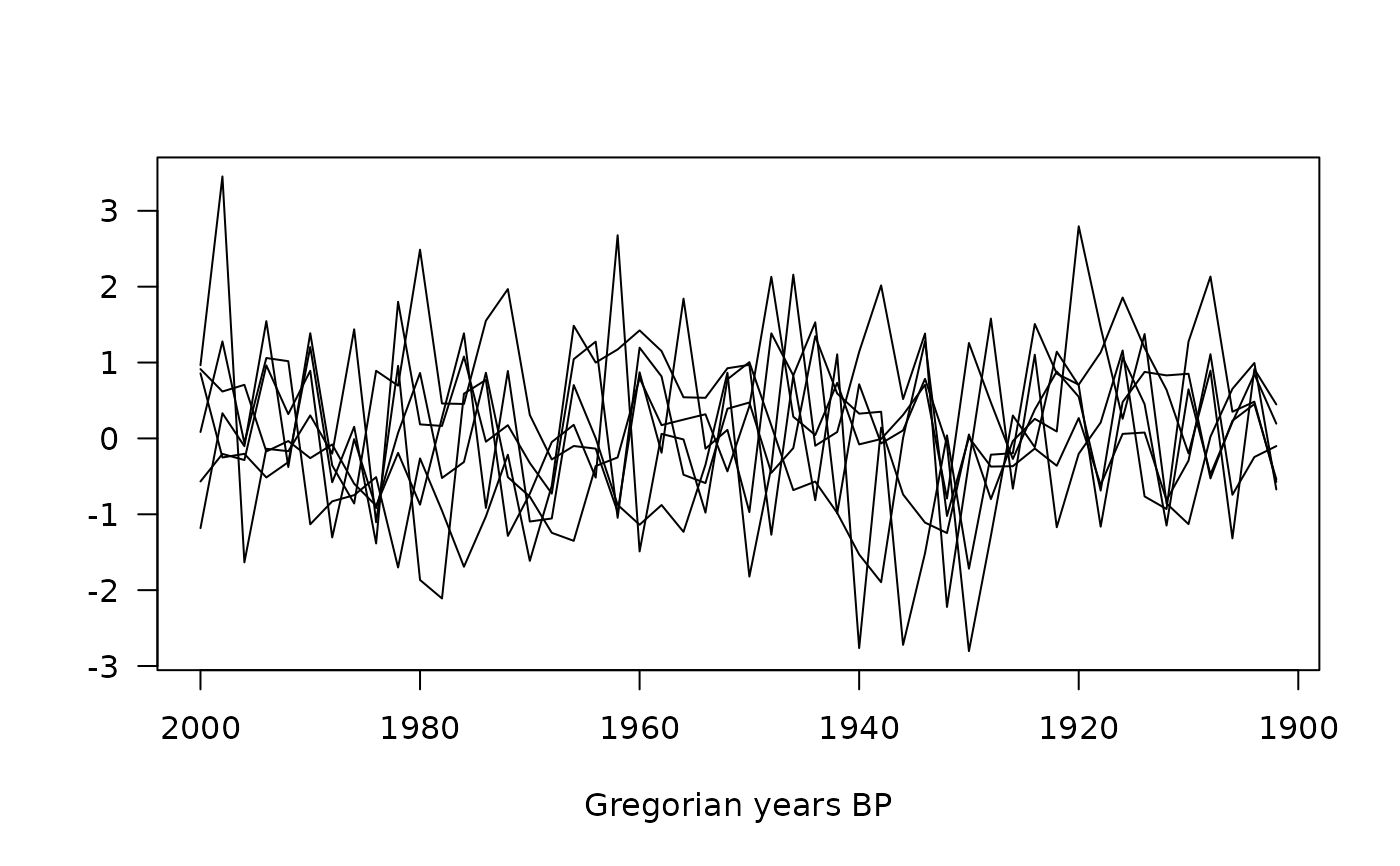## Create 6 x 3 time-series of 50 observations
## Sampled every two years starting from 2000 BP
X <- series(
object = array(rnorm(900), dim = c(50, 6, 3)),
time = seq(2000, by = 2, length.out = 50),
calendar = BP()
)
plot(X, calendar = BP(), flip = TRUE) # BP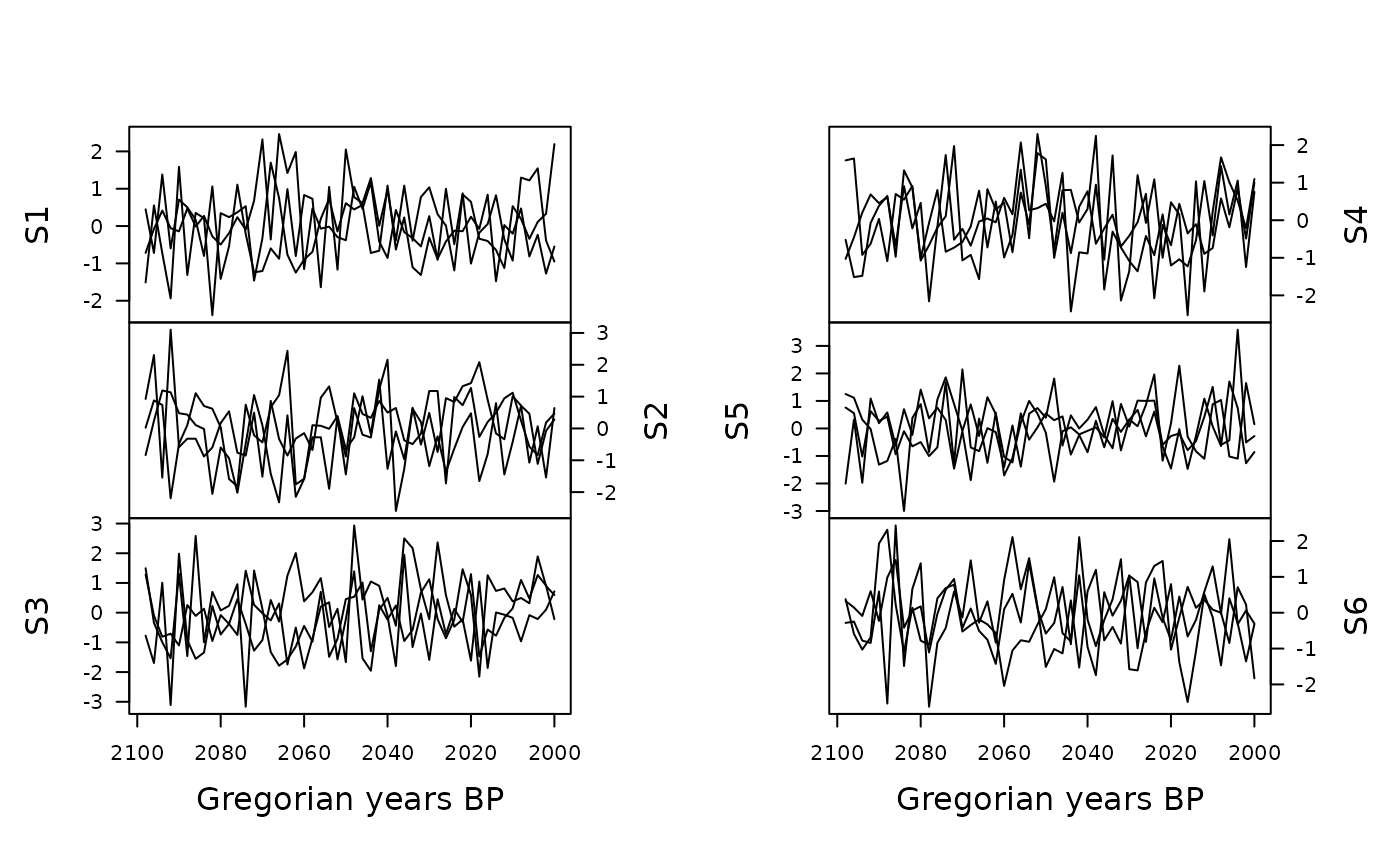plot(X, calendar = b2k(), ncol = 1) # b2k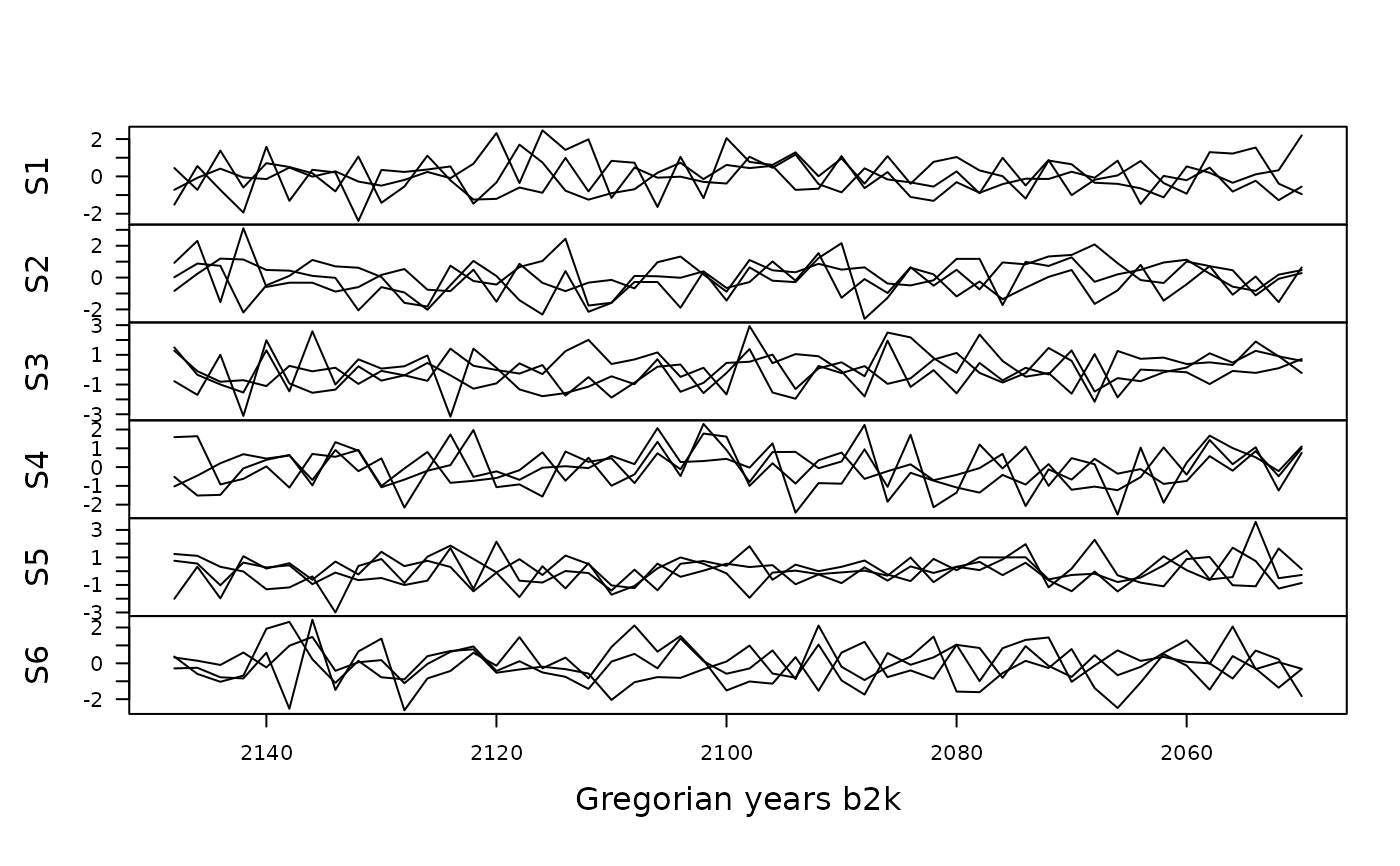## Graphical parameters
plot(X, lwd = c(1, 2, 3), col = c("#004488", "#DDAA33", "#BB5566"))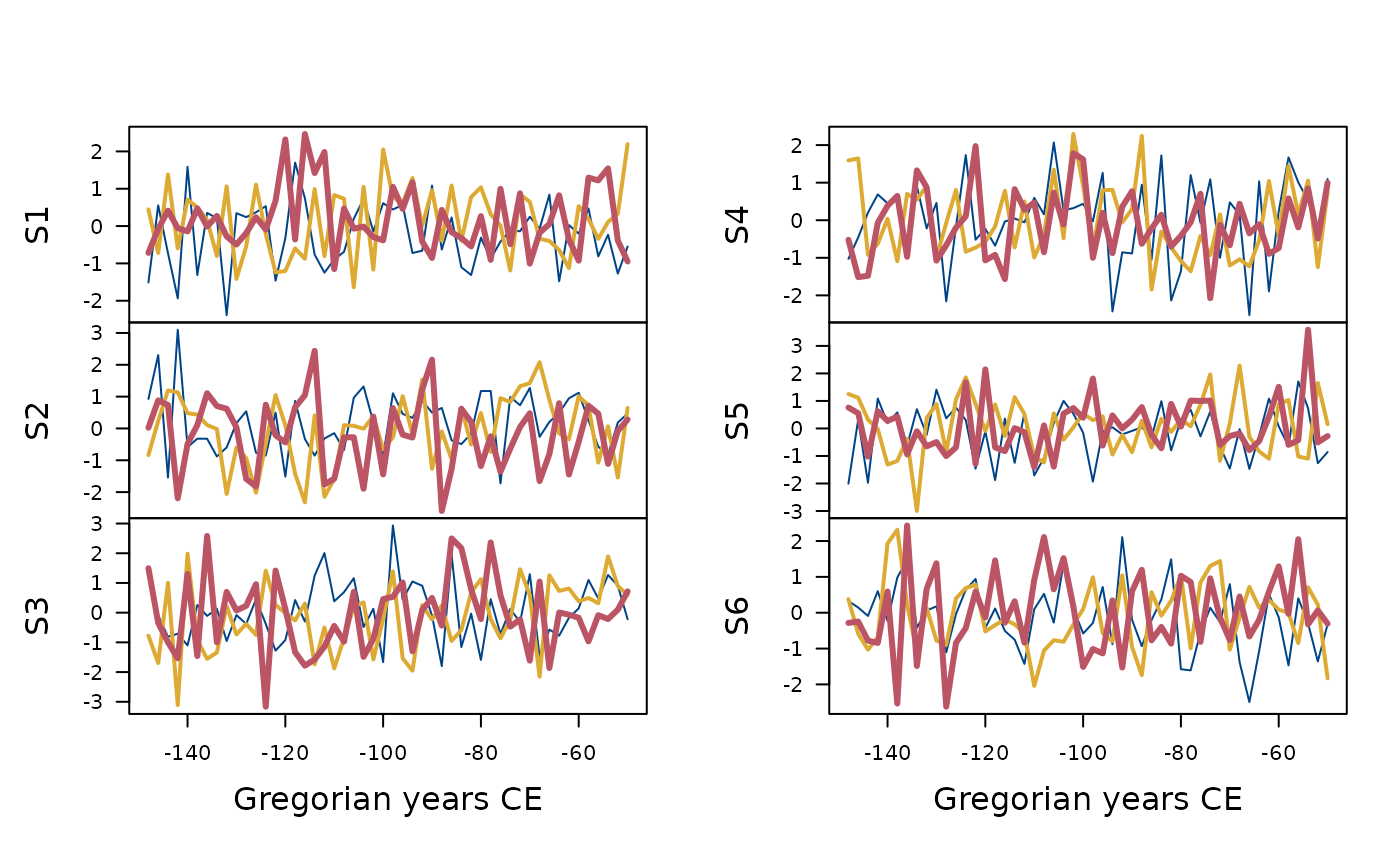plot(X, type = "b", pch = 16, col = c("#004488", "#DDAA33", "#BB5566"))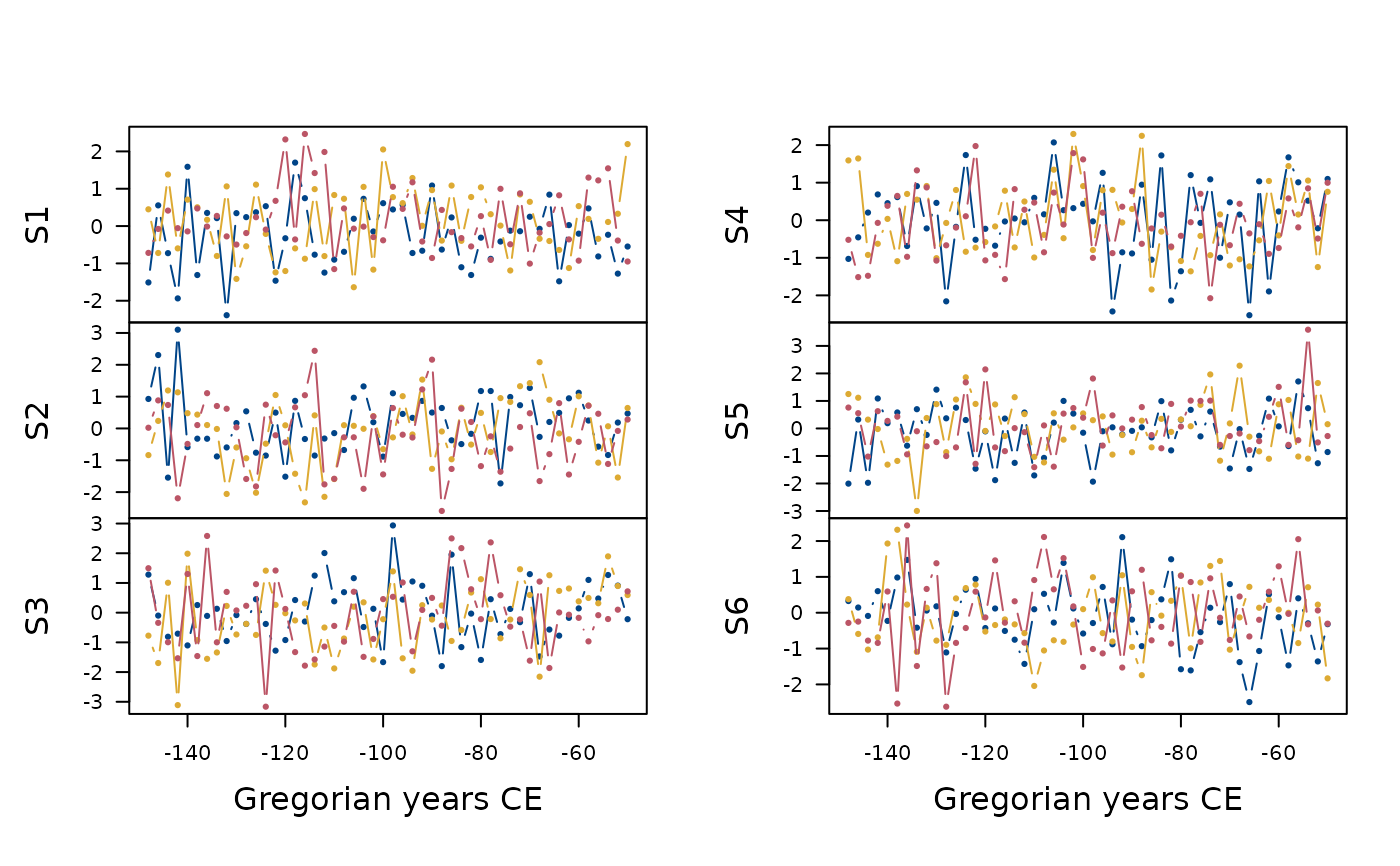plot(X, type = "p", pch = c(16, 17, 18), cex = c(1, 2, 3))